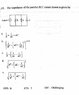# Impedeance of parallel RLC circuit

• hidemi
In summary, the parallel RLC circuit impedance is shown as attached. The equation above is the impedeance of RLC circuit in series, how can I convert that in parallel? Thanks.f

#### hidemi

Homework Statement
The impedance of the parallel RLC circuit shown is given by (as attached)
Relevant Equations
Z = [(R^2 + (XL - Xc)^2)]^1/2
the impedance of the parallel RLC circuit is shown as attached.
The equation above is the impedeance of RLC circuit in series, how can I convert that in parallel? Thanks.

#### Attachments

•1.jpg
27.2 KB · Views: 187
There are duality theorems in linear passive networks like this to literally convert them to a similar system with the same solution with the equivalent of variable substitutions (resistance becomes conductance, inductances becomes capacitance, current becomes voltage, etc.). BUT, it's kind of pointless to go that route for this sort of problem. You can just combine the impedances in parallel. Same as you would do for resistors, except the component values can be complex numbers. This is essentially an algebra problem.

You can use the rule for parallel resistances for this case with the role of resistance played by the complex impedance so it will be $$\frac{1}{Z}=\frac{1}{Z_R}+\frac{1}{Z_C}+\frac{1}{Z_L}$$. As I emphasized these are complex impedances , for example it will be ##Z_R=R## however ##Z_C=\frac{1}{i\omega C}## and ##Z_L=i\omega L##. So you have to do algebra of complex numbers to find the complex ##Z## and then your answer will be the modulus of Z, that is ##\|Z\|##. (The modulus or magnitude of a complex number ##Z=a+bi## is the real number $$\|Z\|=\sqrt{a^2+b^2}$$.)

•hidemi
You can use the rule for parallel resistances for this case with the role of resistance played by the complex impedance so it will be $$\frac{1}{Z}=\frac{1}{Z_R}+\frac{1}{Z_C}+\frac{1}{Z_L}$$. As I emphasized these are complex impedances , for example it will be ##Z_R=R## however ##Z_C=\frac{1}{i\omega C}## and ##Z_L=i\omega L##. So you have to do algebra of complex numbers to find the complex ##Z## and then your answer will be the modulus of Z, that is ##\|Z\|##. (The modulus or magnitude of a complex number ##Z=a+bi## is the real number $$\|Z\|=\sqrt{a^2+b^2}$$.)
Thank you so much.

•Delta2